Field Theory - Online Tutorial 1 - New

by Kevin Tang , new update May 26, 2014

[main pages]

Q1 -

A charge ρl [C/m] is uniformly distributed on the line −a < z < a.   and point P given P (0, b, 0)

Find the following...

* Please review this question, as I didn't solve it fully today ~ good luck :)

* Notice: any BOLD letter means that variable is a VECTOR.

1a) Draw the diagram

1b) Find r, r', r r', | r r' | and dQ.

1c) E at point (0, b, 0)

1a) Draw the diagram..

Solving Time: 3 min

SOLUTION 1a)

Shown in Figure #1 below...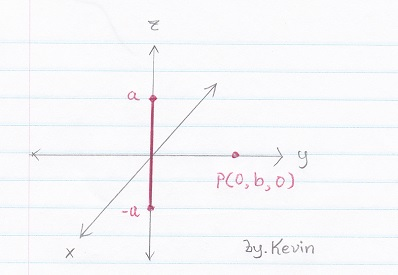Figure # 1

1b) Find r, r', r r', | r r' | and dQ.

Solving Time : 5 min

Let's have fun and give a try...(click the answer that you think it is correct)

Which one is r?

 A r = b Wrong Answer B r = aax + 0ay + 0az Wrong Answer C r = 0ax + bay + 0az Correct! D r = baρ Wrong Answer

Which one is r'?

 A r' = 0ax + 0ay + aaz Wrong Answer B r' = 0ax + bay + z'az Correct! C r' = 0ax + bay + aaz Wrong Answer

Than you can get can get r r' and | r r' |

Next, which one is dQ?

 A dQ = ρl az dz' Wrong Answer B dQ = ρl z' dz' Wrong Answer C dQ = ρl dz' Correct!

SOLUTION 1b)

Answer for r, r', r r', | r r' | and dQ.

Detail solution shown as following (shown in Figure #2)...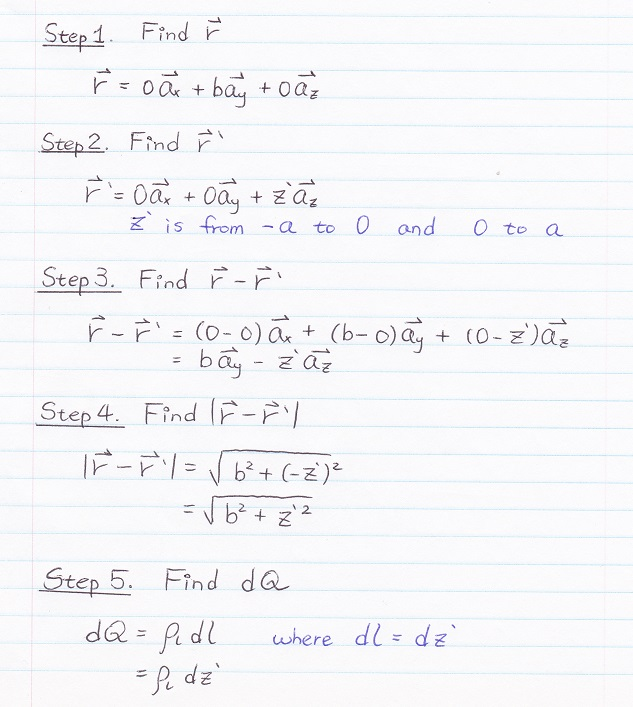Figure # 2

1c) E at point (0, b, 0)

Solving Time : 15 min

SOLUTION 1c)

Actually the solution is much longer than I expected, please take a good look...

First, sub everything into dE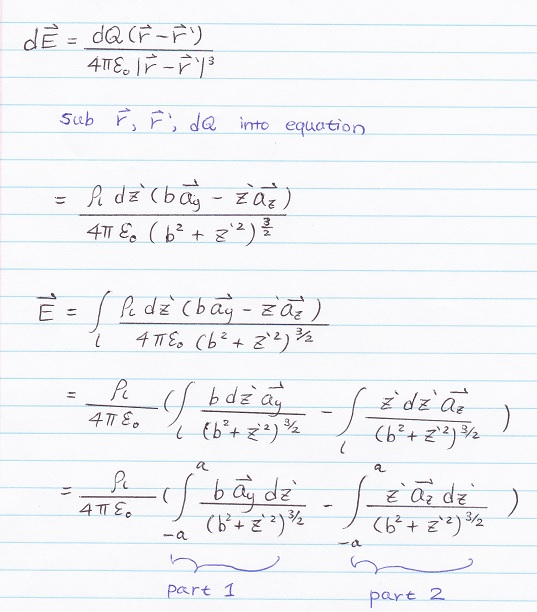Figure # 3

Second, split the integral into 2 PART.

Remember, as I said in class, you can visually see that az part (part 2) will cancel out to zero due to symmetric,

however, here I am still going to show you how its lead to zero.

Now, we start with ay part (part 1) of the integral...(shown in Figure #4)

you need to applied integral by trigonometric substitution (or you can directly check it on Integral Table)Figure # 4

.

.

.

.

Part 2, az part of integral, its will cancel out and lead to zero as shown following (shown in Figure #5)...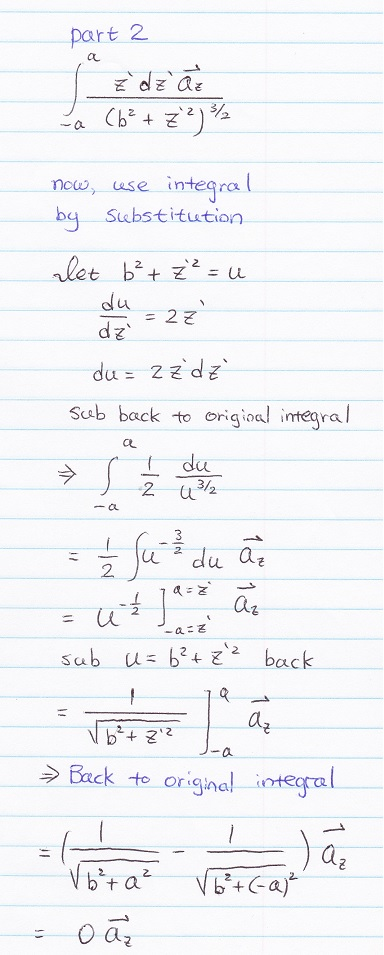Figure # 5

Last step, sub everything into E, and you will get the final answer (shown in Figure #6) :)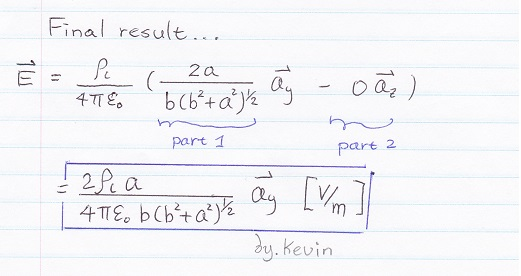Figure # 6

You may leave me any message if you have any questions, I will try to answer ASAP, thanks and good luck :)